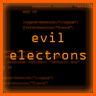|

# M-PSK Modulation

Uptill now, we have discussed the basic concepts of digital modulation techniques.Let’s move one step ahead!
In digital modulation two commonly used terms are Symbols n Modulation order(M) Symbol:
It is actually a combination of bits.For instance, So and S1 are two symbols.Where ,So=00,S1=11 etc
Modulation order-M:
It refers to the number of states used in modulation,or the number of symbols.If M=2 it means two distinct states are being used in modulation. States could be different frequencies, amplitudes, or phase angles.If M is represented in power of ‘2’, that shows the number of bits per symbol.
For M=4
There will be 4 symbols(S0, S1, S2, S3).4=2^2.Therefore ,each symbol will be represented by Two bits.S0=00,S1=01,S2=10,S3=11
Keeping in view the ‘M’ factor, let’s restate the modulations techniques as:
M-PSK:
For M=2: It is called as BPSK,(where B stands for Binary).Number of symbols are two. S0 and S1.As 2=2^1, so each symbol is represented by a single bit, therefore we wil have, S0=0 and S1=1
For M=4: It is called as 4-PSK.Number of symbols are Four.S0,S1,S2,and S3.As 4= 2^2,so each symbol is a combination of two bits,so we will have,S0=00,s1=01,s2=10,s4=11
So on and so forth for M=8,16,32,64
As PSK is phase modulation ,therefore the number of states actually refer to distinct Phase angles.These phase angles are used to modulate the carrier.

For M=2 , we have 2 phases/states
Total angle is 360 degree
360/M =180 deg
So.’S0’ will b represented by 0 degree and ‘S1’ will be represented by 180(phase difference of 180 between symbols).That is, if 0 bit appears in signal, it will modulate the carrier wave with 0 degree, and 1 bit will modulate the carrier with 180 deg phase.
For M=4, we have 4 distinct phases
360/4 =90
So, Symbols will be represented as:
S0=0 deg,S1=90 deg,S2=135 deg,S3=180 deg
As we have the following symbols ,S0=00,s1=01,s2=10,s4=11.So, if 00 bits appear in signal, they will modulate the carrier wave with 0 degree,01 will modulate the carrier with 90 deg phase.10 will modulate the carrier with 135 deg n 11 will modulate it with 180 deg.
For M=8,we have 8 phases
360/8=45 deg
So,So=0deg,S1=45 deg,S2=90 deg,S3=135 deg,S4=180 deg,S5=225 deg,S6=270deg deg,S7=360 deg
Each symbol is represented by three bits here,
S0=000,S1=001,S2=010,S3=011,S4=100,S5=101,S6=110,S7=111
So, 000 bits will indicate 0 degree phase shift ,001 will represent 90 deg phase.010 will modulate the carrier with 135 deg ,0 11 will refer to 180 deg, n so on .
Similarly, for M-FSK, M frequencies are used to modulate a carrier and for M-ASK , M amplitude levels will be used.Posted by Sadaf Dilshad Khan on 18:04. Filed under , , . You can follow any responses to this entry through the RSS 2.0. Feel free to leave a response